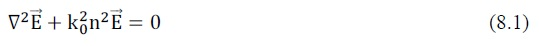For wave propagating in periodic structures made of semiconductor material, the build-in grating or corrugation induces dielectric or refractive index perturbation, and such perturbation results in an interaction between the forward and backward propagating waves. Historically, various
approaches like coupled wave theory  and Bloch wave analysis  have been adopted. Although these methods were proven to be equivalent , researchers have been keen on coupled wave theory because it is easier to understand, and because numerical algorithms can be implemented to solve the equations  .
In a homogeneous, source-free and lossless medium, any time harmonic electric field must satisfy the vector wave equationwhere the time dependence of the electric field is assumed to be, n is the refractive index andis the free space propagation constant.
In a semiconductor laser which has a transversely and laterally confined structure, the electric field must satisfy the one-dimensional homogeneous wave equation such thatConsider a multi-dielectric stack in which periodic corrugations are formed along one boundary as illustrated in Fig 8.1.Figure 1 General multi-dielectric layers used to show the perturbation of refractive index and amplitude gain. QE(R) and QF(R) are two corrugated functions.